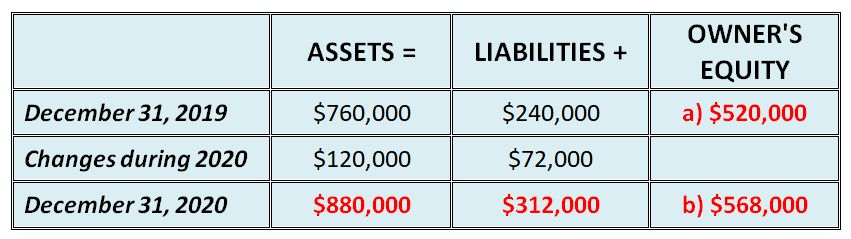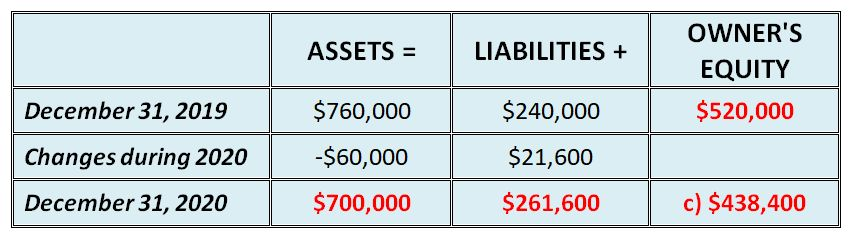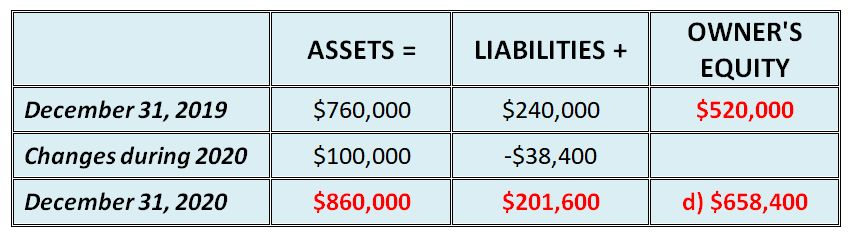# Accounting Equation Questions and Answers

by Kevin
(North Carolina, USA)

Before you begin: For purposes of exams and testing it's important to make sure you not only get the questions right but are completing them at the right speed. Use a watch or clock to time yourself for this exercise.

Difficulty Rating:
Beginner

Time limit:
10 minutes

## Question:

Tom Jones is the owner and operator of Jones Enterprise, a motivational consulting business. At the end of its accounting period, December 31, 2019, Jones Enterprise has assets of \$760,000 and liabilities of 240,000.

Use the accounting equation to calculate the answers in each of the following:

a) Tom Jones, capital, as of December 31, 2019

b) Tom Jones, capital, as of December 31, 2020, assuming that assets increased by \$120,000 and liabilities increased by \$72,000 during 2020

c) Tom Jones, capital, as of December 31, 2020, assuming that assets decreased by \$60,000 and liabilities increased by \$21,600 during 2020

d) Tom Jones, capital, as of December 31, 2020, assuming that assets increased by \$100,000 and liabilities decreased by \$38,400 during 2020

e) Net income during 2020, assuming that as of December 31, 2020, assets were \$960,000, liabilities were \$156,000, and there were no additional investments or withdrawals.

## Solutions:

a) and b):To get the answer for a) above, we simply use the basic accounting equation.

ASSETS = LIABILITIES + OWNER'S EQUITY

If we turn this around to make owner's equity the subject, then:

OWNER'S EQUITY = ASSETS - LIABILITIES

So we take the assets and minus the liabilities.

In other words, \$760,000 - \$240,000 =
\$520,000

For b) we first add the changes to assets and liabilities to get their new balances. Then we work out the new balance of owner's equity in the same way: \$880,000 - \$312,000 =
\$568,000

The answers below for c) and d) basically work in the exact same way - get the new balance of assets and liabilities and then use these new balances to work out the new balance of equity.

c):d):e):This last part (e) is a bit trickier than the previous ones.

What you have to do is first calculate the new balance of owner's equity.

The net income (also simply known as net profit) is the change for the year, and is the difference between the old and new balances of owner's equity.

In other words, \$804,000 - \$520,000 =
\$284,000

And there you have it! Hope you enjoyed our accounting equation questions and answers!

- Michael Celender
Founder of Accounting Basics for Students

Related Questions & Tutorials:
Return from Accounting Equation Questions and Answers to our main page of Basic Accounting Questions and Answers

 Excellent by: Ms Matlenya student at Richfield graduate Am a first year student with no background knowledge on accounting but the exercises gave me an idea on the accounting basics, thank you!

 edsd by: thevine this is easy to for reference but its not clearly mentioned as noticed

 accounting equation by: Anonymous really enjoyed the equation.

 WHOO by: Anonymous Whoo accounting is fun it like a game to me ...it is fun......lol